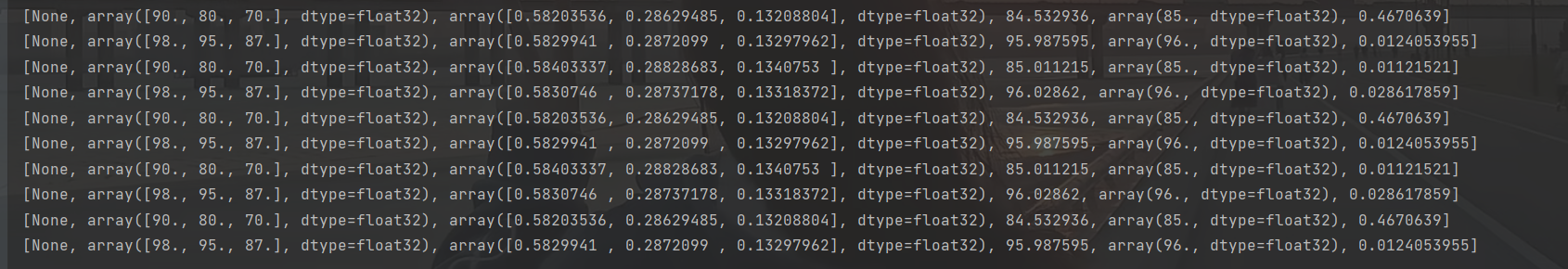# 深度学习—三好学生各成绩所占权重问题（3）

🔝🔝🔝🔝🔝🔝🔝🔝🔝🔝🔝🔝

🥰 博客首页：knighthood2001

😗 欢迎点赞👍评论🗨️

❤️ 热爱python，期待与大家一同进步成长！！❤️

👀给大家推荐一款很火爆的刷题、面试求职网站👀

之前，我们从三好学生成绩问题开始，设计出了解决该问题的神经网络模型，但是这个模型更多的是从一般的思维方式来设计的，这与神经网络通常设计中的思路并不一致，因此本文章，我们来看看如何优化该模型，让它的逻辑更清晰、运行更高效。

# 全部代码

``````import tensorflow as tf

tf.compat.v1.disable_eager_execution()

x = tf.compat.v1.placeholder(shape=, dtype=tf.float32)
yTrain = tf.compat.v1.placeholder(shape=[], dtype=tf.float32)

w = tf.Variable(tf.zeros(), dtype=tf.float32)

n = x * w

y = tf.reduce_sum(n)

loss = tf.abs(y - yTrain)

optimizer = tf.compat.v1.train.RMSPropOptimizer(0.001)

train = optimizer.minimize(loss)

sess = tf.compat.v1.Session()

init = tf.compat.v1.global_variables_initializer()

sess.run(init)

for i in range(5000):
result = sess.run([train, x, w, y, yTrain, loss], feed_dict={x: [90, 80, 70], yTrain: 85})
print(result)

result = sess.run([train, x, w, y, yTrain, loss], feed_dict={x:[98, 95, 87], yTrain: 96})
print(result)``````

# 代码讲解

之前设计的神经网络模型中，把学生的德育、智育、体育3项分数分别对应x1、x2、x3这了个输入层的节点，这样本身没有问题，但是假设又增加了一个艺术分数， 那么就需要在输入层增加一个x4节点，在隐藏层对应的也要增加一个n4节点。也就是说，输入数据改变时，即使整套逻辑没有变，也要去修改整个网络模型，比较麻烦。

另外，节点数多了也会让模型图看起来比较复杂。所以在通常的神经网络中，很多时候会把这种串的数据组织成一个 “向量”来送入神经网络进行计算。这里的“向量”与数学几何中的向量概念稍有不同，就是指一串数字，在程序中用一个数组来表示，例如，三好学生成绩问题中第一个学生的3项分数可以用[90, 80, 70]这样一个数组就表示出来了。数组是有顺序的，可以约定第一项代表德育分、 第二项代表智育分、 第三项代表体育分。向量中有几个数字，一般就把它叫作几“维”的向量，例如刚才这个向量就是一个三维向量。

``````x1 = tf.compat.v1.placeholder(dtype=tf.float32)
x2 = tf.compat.v1.placeholder(dtype=tf.float32)
x3 = tf.compat.v1.placeholder(dtype=tf.float32)``````

``x = tf.compat.v1.placeholder(shape=, dtype=tf.float32)``

变量x的定义语句稍有不同，其中增加了一个命名参数shape,这是表示变量x的形态的，它的取值是“”， 表示输入占位符x的数据将是一个有3个数字的数组，也就是一个三维向量。

w1、w2、w3这3个可变参数也被缩减成了一个三维向量w:

``w = tf.Variable(tf.zeros(), dtype=tf.float32)``

yTrain因为只是一个普通数字，不是向量，如果要给它个形态的话， 可以用一个空的方括号“[]”来代表。

``yTrain = tf.compat.v1.placeholder(shape=[], dtype=tf.float32)``

``````n = x * w
``````

``y = tf.reduce_sum(n)``

tf.reduce sum函数的作用是把作为它的参数的向量(以后还可能会是矩阵)中的所有维度的值相加求和，与原来y=n1 +n2 + n3的含义是相同的。

``````    result = sess.run([train, x, w, y, yTrain, loss], feed_dict={x: [90, 80, 70], yTrain: 85})
print(result)

result = sess.run([train, x, w, y, yTrain, loss], feed_dict={x:[98, 95, 87], yTrain: 96})
print(result)``````

# 结果展示看最后的几条结果，我们可以看到，误差也被控制到很小的范围，3个权重也分别接近预期值。